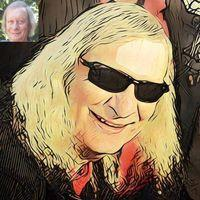BrainDen.com - Brain Teasers# rocdocmac

Members

431

• #### Days Won

15

rocdocmac had the most liked content!

## Reputation

10
1.## Math Challenge Puzzle: Fill the white square boxes such that the equations are satisfied

• 1
•2. Nothing wrong with the "formula" in red! The distance here is expressed in parsec (pc), where 1 pc ~31 trillion km.
3. For interest's sake, if no true fractions were involved at all, there is an alternative solution ...
4. YAY! second number: the last digit of powers of 3 runs through the cycle (3971), with exponent divisible by 4 ending in 1. 556677 is 1mod4 so it ends in 3.
5. Earlier today (whilst attending his birthday party) I asked Andy his age, but he said that I can figure that out easily by myself! All I had to do was to add four numbers together. The first of these four numbers is the last digit in the figure obtained when 2 is raised to the power of 667788 (2667788). Similarly, I had to find the last digit in the evaluation of 3556677, 7445566, and 8334455 to complete the sum. If today is the 12th of March 2020, when was Andy born?
6. Ah-ha! The first toss is irrelevant. Thus the second player has the best chance ...
×

• #### Activity

• Riddles
×
• Create New...